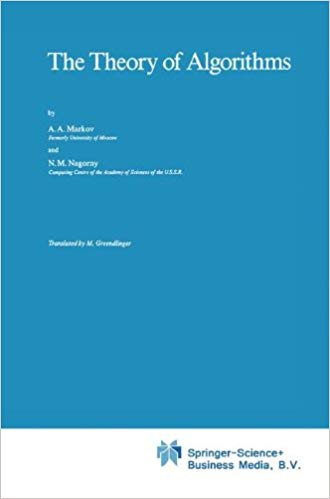@devilogic 2019-03-10T09:07:26.000000Z 字数 5752 阅读 808

# 计算机程序设计艺术研修笔记（1.1 算法）

TAOCP

1. 算法(algorithm)一词的由来
2. 算法的使用集合论的一个表示
3. 算法的一个限制表示

$\quad$这里有趣的是使用集合论的形式来定义计算，以及对于涉及仅是某种初等运算的举例表示，从中可以看出从一个数学家的角度来思考事物，作者从日常对象中进行抽象并形成理论，这是科学家的一种思考方式，将具体的对象抽象化、系统化。将简单变复杂是科学家的想法。工程师的角度愿意将复杂的问题变简单，便于实现。

$\quad$以上是摘自第一卷的第7页,主要是将算法这一个抽象概念符号化的一个表示。这里还比较容易理解，就是用集合表示算法，难理解的是这句：“注意，如果$x_k$$\Omega$中，则$x_{k+1}$也是。因为在这样的情况下，$x_{k+1}=x_k$。”。这里应该是指得算法的收敛性。在之前作者提出了算法所要具备的五个特征：

1. 有限性
2. 确定性
3. 输入
4. 输出
5. 能行性

$\quad$这里的$f(q)$应等于$q$指得是通过$f$计算得到的值也在同一空间内，有域的概念在里面。

$\quad f((\delta, j)) = (\delta, a_j)$，如果$\theta_j$不在$\delta$中出现；
$\quad f((\delta, j)) = (\alpha \phi_j \omega, b_j)$，如果$\alpha$是使$\delta=\alpha \theta_j \omega$最短的串；
$\quad f((\delta, N)) = (\delta, N)$

$\quad$以上指明了是初等运算，这里可以理解为线性运算，非线性的组合应该会使得结果不在定义的计算机状态全集$Q$内，而$A^*$表示了通过初等运算$f$可以组成的所有得到的状态。$(\delta, j)$这个二元组，$\delta$表示当前的计算机状态，而$j$应该是表示算法运行到第几次。$I$$j=0$的时的计算状态，也就是初始状态，而$\Omega$表示$j=N$的计算状态也就是算法结束时的状态，也就是输出。随后定义了一个算法的完成状态就是定义的最终输出$\delta$是由目标状态$\theta$以及$\alpha$$\omega$的一个组合来表示。最后定义了三种算法的结果。一、算法正常运行，但是未找到有效解。二、算法正常运行，找到有效解。三、算法遍历完所有的参数空间(这里由$N$的步数来指代)但是未找到解。
$\quad$可以看出所有大师的一个思想方式，将具体的事物进行抽象，抽象之后进行符号化，从而可以使得事物可以满足某种运算规律，最后形成一套系统。
$\quad$具体事物->抽象->符号化->可计算性->新的系统，这也是有特殊到一般的要给过程。

#### 习题

$\quad 1.$ 正文中说明了如何使用替代符号，通过设置$t \leftarrow m$$m \leftarrow n$$n \leftarrow t$来交换变量$m$$n$的值。说明如何通过一系列的替代，把四个变量$(a,b,c,d)$的值重新安排成$(b,c,d,a)$。换言之，$a$的新值是$b$原来的值，等等。试使用最少的替代次数。

$\quad$$t \leftarrow a$$a \leftarrow b$$b \leftarrow c$$c \leftarrow d$$d \leftarrow t$

$\quad 2.$ 证明，在步骤$E1$开始时，$m$总是大于$n$的，除了这一步头一次出现时可能出现相反情况外。

$\quad \mathbf E1.$[求余数] 以$n$$m$并令$r$为所得余数。(我们将有$0 \le r \lt n$。)
$\quad \mathbf E2.$[余数为零?] 若$r=0$，算法结束，$n$即为答案。
$\quad \mathbf E3.$[减少] 置$m \leftarrow n, n \leftarrow r$，并返回步骤$\mathbf E1$

$\quad$$\mathbf E1$中如果$m \le n$，如果这里的等号成立，那么余数$r=0$，算法结束，如果是$n \gt m$的情况，则它们的余数$r$$m$本身，进入到$\mathbf E3$中，$m \leftarrow n$$n \leftarrow r$, 其中$m = r$, 相当于在$\mathbf E3$中完成了交换。
$\quad$$\mathbf E1$中如果$m \gt n$，根据余数必定小于除数的定理。则在$\mathbf E3$中永远保证了$m \gt n$的交换。

$\quad 3.$ (为了提高效率)改变算法$E$使得像$"m \leftarrow n"$这样平凡的替代运算都加以避免。以算法$E$的风格来写出这个新算法，并称之为算法$F$

$\quad \mathbf F1.$[求余数] 以$n\%r$并令$n$为所得余数。(我们将有$0 \le n \lt r$。)
$\quad \mathbf F2.$[余数为零?] 若$n=0$，算法结束，$r$即为答案。
$\quad \mathbf F3.$[求余数] 以$r\%n$并令$r$为所得余数。(我们将有$0 \le r \lt n$。)
$\quad \mathbf F4.$[余数为零?] 若$r=0$，算法结束，$n$即为答案。
$\quad \mathbf F5.$[跳转到求余数] 跳转到流程$\mathbf F1$

$\quad 4.$ $2166$$6099$的最大公因子是多少?

$\quad gcd(2166,6099) = 57$

$\quad 5.$ 证明出现前言后的“阅读本套书的流程”，按我们给出的五条准则的三条，实际上不是一个真正的算法！并指出它和算法$E$之间格式上的差别。

$\quad$本书给出了算法的五项特性：一、有限性，二、确定性，三、输入，四、输出，五、能行性。

$\quad$阅读流程并不是有限的，因为并无一个明确退出算法的步骤。因为无法合理的评估阅读这一事件的完成的具体时间，因此它并非确定的。其次阅读流程并不一个明确的输入与输出。由于无明确退出的步骤，以及无法评估具体月底某节的具体时间，那么并不能通过纸笔进行手工运算来确定算法，因此它是不可行的。综上所述本书的阅读流程并不是一个真正的算法。

$\quad 6.$$n=5$时，执行步骤$\mathbf E1$的平均次数$T_5$是多少?

$\quad$平均次数$T_5$就是遍历$m=1,2,\ldots$的全部情况后除以当前遍历的次数。根据同余定理，这里当$m \gt n$后会形成一个循环。$1 \% 5 = 11 \% 5$，所以当$m=1$$m=11$时，$\mathbf E1$的执行次数都相同。这里仅需要遍历$m=1,2,3,4,5$即可。而$1,2,3,4$的取模与$6,7,8,9$的次数一致。这里可以得到一组运行的平均次数：

$\quad$无论$m$有多大，我们都可以将它以$n$进行分组。由于$m=qn+r$，所以这里自然的分为$q$组。最后的余数$r$可以想想它的值是小于$n$的，但是这里的$r$是不确定，除去最后整除的数字。最多超不过$\frac{2+3+4+3}{4}=3$。综上所述我们可以得出当$n=5$的平均运行次数的上下限：

$\quad$这里使用$a$来替代$2.6$$b$来替代$3$，那么在某个固定数$n$下，其通解形式为:

$\quad 7.[M21]$ 假设$m$已知而允许$n$取遍所有的正整数；令$U_m$是在算法$E$中执行步骤$\mathbf E1$的平均次数。证明$U_m$是明确定义的。$U_m$$T_m$有什么关系吗？

$\quad$题目中所说的$U_m$是明确定义的，这里理解为是固定的。不会出现值不确定的情况。

$\quad$这里分为两个阶段来讨论。当$n$取遍$1,2,\ldots,m$的过程中，$m \ge n$，当$n$$m+1,m+2, \ldots$$m \lt n$
$\quad$$m \ge n$时，这里需要考虑两种情况，一种是$m$可以被$n$整除，一种是不可以。前者的平均次数为$1$，后者相当于当$m \lt n$时题目$6$中的$T_n$，但是排除了整除的情况，所以这里$2 \le U_m \le a_n+b_n \frac{r_n}{r_q}$，其中的$a_n,b_n,r_n,r_q$表示在$n$固定下的取值。综上所述，当$m \ge n$时：

$\quad$$m \lt n$时，也分为两种情况，一种是$m$可以被$n$整除，一种是不可以。那么前者的平均次数为$1$，而后者由于$m \lt n$，则$\mathbf E1$必执行一次，在$\mathbf E3$中进行交换，同理$2 \le U_m \le a + b\frac{r}{q}$
$\quad$综合以上两种情况$U_m$$T_n$的平均次数是一样的。固定一方，取另外一边的所有正整数情况。都会出现一方比另外一方：等于三种情况。如果出现除数大于被除数而在$\mathbf E3$都会进行交换。所以两者没有区别，但是由于一开始$m \gt n$的，所以必然会执行$\mathbf E3$一次。所以：

$\quad 8.[M25]$ 通过描述等式$(3)$中的$\theta_j,\phi_j,a_j,b_j$，给出计算正整数$m$$n$的最大公因子的一个“能行的”形式算法。令输入由串$a^mb^n$表示，即$m$$a$后边跟着$n$$b$。试尽可能简单地给出你的解。[提示：使用算法$E$，但替代步骤$\mathbf E1$中的除法，置$r \leftarrow |m-n|，n \leftarrow min(m,n)$。]

$\quad$这道题感觉是要探讨整数的素数分解的算法。从题目所说的：“令输入由串$a^mb^n$表示”这句话来看。整数分解有非常多的算法，而现代密码学的基础也是基于大整数难以分解的基础上。我之前写过一篇对最新素数分解算法AKS分析的文章玩命的销售日志 2017.10.23。这篇论文证明了在多项式时间下可以完成对大整数的分解并提出了一套基于多项式分解的算法。
$\quad$这道题我确实不知道应该如何下手，主要是理解不了题目的意思。所以这边翻看了答案，帮助理解题意。

$A = \{a,b,c\},N=5$。这个算法将以字符串$a^{gcd(m,n)}$结束。

$\quad$让我们从答案来理解一下题意。

$\quad$给定两个正整数$m$$n$，求它们得最大公因子，即能够同时整除$m$$n$的最大正整数。
$\quad \mathbf E1.$[求余数] $r \leftarrow |m-n|，n \leftarrow min(m,n)$。(我们将有$0 \le r \lt n$。)
$\quad \mathbf E2.$[余数为零?] 若$r=0$，算法结束，$n$即为答案。
$\quad \mathbf E3.$[减少] 置$m \leftarrow n, n \leftarrow r$，并返回步骤$\mathbf E1$

$\quad 9.[M30]$ 假设$C_1=(Q_1,I_1,\Omega_1,f_1)$$C_2=(Q_2,I_2,\Omega_2,f_2)$是计算方法。例如：$C_1$可代表等式$(2)$中的算法$E$，但$m$$n$在数量上要加限制，而$C_2$可代表实现算法$E$的计算机程序。（因此$Q_2$可以是机器的所有状态的集合，即它的内存和寄存器所有可能的配置；$f_2$可以是单个机器动作的定义；$I_2$可以是初始状态，包括确定最大公因子的程序以及$m$$n$的值。）
$\quad$试表述“$C_2$$C_1$的表示”或“$C_2$模拟$C_1$”的概念的集合论定义。这在直观上意味着$C_1$的任何计算序列都由$C_2$加以模拟，除$C_2$在计算中采用更多的步骤以及在它的状态中它可能保留更多的信息外。（我们因此得到对于“程序$X$是算法$Y$的一个实现”这一语句的一个严格的解释。）

#### 涉及的书籍The Theory of Algorithms• 私有
• 公开
• 删除